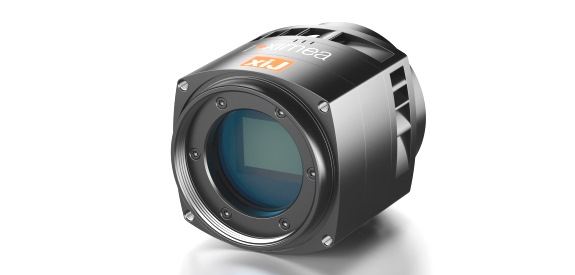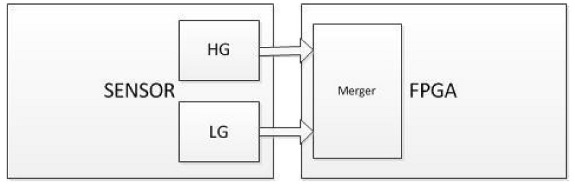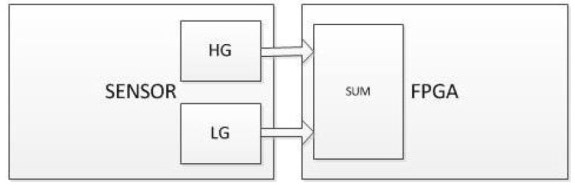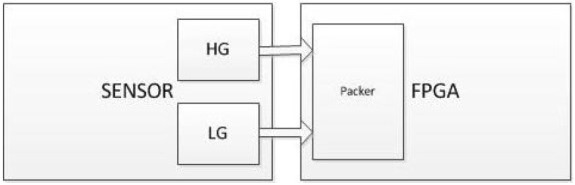Support» Knowledge Base » ADC - Bit readout explained

# Support¶

### Contact Support¶` `
` `

Brochure

` `
` `

Readout from sCMOS (Scientific CMOS) sensors used in XIMEA cameras is a bit more complicated which is why this simple explanation can help understand the final speed (frames per second) and output:

## For PCI Express cameras - CB042 models¶

### Rolling shutter¶

Sensor readout 2 x 12 bit means one High gain (HG) and one Low gain (LG) channel.
That is how these sensors work.
` `
Transport depends on the output_bit_depth parameter.
It can be set to 8, 10, 12, 16 which means 2x8, 2x10 and so on.
` `
Image formats:
RAW8, RAW16 - data are merged from HG and LG.
RAW8x2, RAW16x2 - RAW data from the sensor. Both HG and LG data for each pixel are present.

### Global shutter¶

Sensor readout 4 x 10 bit means again High gain and Low gain data, but for Signal image and Reset image each.
` `
Transport depends on output_bit_depth parameter.
It can be set to 8, 10, 12, 16 which means 4x8, 4x10 and so on.
` `
Image formats:
RAW8, RAW16 - data are merged from HG and LG, and both Signal and Reset images.
RAW8x4, RAW16x4 - RAW data from the sensor. Both HG and LG data for a pixel for Signal image and Reset image are present.

### Calculator¶

To calculate the frame rate and other parameters you can use this helpful TOOL.
Fill inputs (the orange values) and see the expected results.
Each input is connected to a xiApi parameter - e.g. Transport bit depth field is XI_PRM_OUTPUT_DATA_BIT_DEPTH xiapi parameter
Because of the processes mentioned above, in this case, if you change the Transport Bit Depth from 8 to 10 the Frame rate is still the same for example.
` `
Note: 1300 MB limit was introduced into the Calculator because not all computer configurations were able to support higher speed, but now certain PCs could reach stable 1750 MB.

## For USB3 + sCMOS cameras - xiJ models¶

Gpixel sensors (GSENSE400, GSENSE2020, GSENSE5130 and others) offer 3 operation modes:
HDR, STD and CMS
These sensors have two on-chip 12bit ADCs.
First, high gain ADC (HG) is optimized for low readout noise.
Second, low gain ADC (LG) is optimized for full well capacity.

### 1. HDR.¶Merger:
` `
` `

O16 = (DL12 * k + DH12 * (1 – k) * g) * 16 = DL12 * k * 16 + DH12 * (1 – k) * g * 16

` `
` `

there:

` `
` `

O16 – 16bit output signal.
DL12 - 12bit data of LG channel.
DH12 - 12bit data of HG channel.
k = DH12 / 4095.
g = gL / gH.
gL – gain of LG channel.
gH – gain of HG channel.

` `
` `

In HDR mode the analog gains are fixed (gL and gH) and can’t be changed by API.

### 2. CMS mode.¶SUM:

` `
` `

O16 = (DL12 + DH12) * 8 = D13 * 8

` `
` `

gL = gH
Both ADCs readout the same pixel at one time.

### 3. STD mode.¶Packer:

` `
` `

O12 (pixel 0) = DL12 (pixel 0, row 0)
O12 (pixel 1) = DH12 (pixel 0, row 1)
O12 (pixel 2) = DL12 (pixel 1, row 0)
O12 (pixel 3) = DH12 (pixel 1, row 1)

` `
` `

gL = gH
In CMS and STD modes gain on both channels can be changed in API.

## For large sensor and pixel cameras - MX377 models¶

Here you can see a table with the preliminary image quality parameters for different modes:

` `
` `

 Modes gain [dB] dark noise [e] FWC [ke] Dyn.Range [dB] HDR_LG -- 9.8 130 82.45 CMS 0 21.5 115 74.57 CMS 25 2.65 7.6 69.15 STD 0 26 105 72

` `
` `

Full HDR mode is also planned which will have lower dark noise (as compared to HDR_LG mode) while leaving the FWC same.

` `
` `